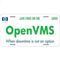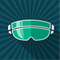Operating System - OpenVMS

# Access ViolationVisitor

## Access Violation

We have a multi-threaded application written in Pro*C (C with embedded SQL) to FTP files to various locations.  This was running successfully for several years in a Alpha server running Oracle 10.1  In May this year, we moved to Itanium running on Oracle 11.2.  The FTP server which runs continuously under Application Process Manager (APM), time to time crashes and the APM successfully restarts it.  It spools out three kind of errors. Most common pasted below.  At your request, I will provide more information.  The common stack dump pasted below.

Thank you for your assistance.

Regards

James

%DCL-I-SUPERSEDE, previous value of SYS\$INPUT has been superseded
%SYSTEM-F-ACCVIO, access violation, reason mask=00, virtual address=000000000000003A, PC=FFFFFFFF80A36891, PS=0000001B

Improperly handled condition, image exit forced.
Signal arguments: Number = 0000000000000005
Name = 000000000000000C
0000000000000000
000000000000003A
FFFFFFFF80A36891
000000000000001B

Register dump:
R0 = 0000000000000000 R1 = FFFFFFFFCB358C00 R2 = 0000000000000239
R3 = 0000000000000061 R4 = 0000000007536780 R5 = 0000000007535F30
R6 = 0000000000000000 R7 = 0000000000000000 R8 = 0000000000000001
R9 = 0000000007535F30 R10 = FFFFFFFFCB1CDED0 R11 = FFFFFFFFCB4E0000
SP = 0000000007535E30 TP = 0000000007551200 R14 = 0000000000000004
R15 = 00000000000004FC R16 = 0000000007536785 R17 = 0000000000000054
R18 = 000000000000003A R19 = 0000000007535E28 R20 = FFFFFFFFCB2D9580
R21 = 0000000000000000 R22 = 0000000000000010 R23 = 0000000007535DFC
R24 = 0000000007535DE0 R25 = 0000000000000003 R26 = FFFFFFFFCB2E1088
R27 = 0000000007535DD8 R28 = 000000000000003A R29 = 0000000007535E30
R30 = 0000000007535C78 R31 = FFFFFFFF80A35F60 PC = FFFFFFFF80A36891
BSP/STORE = 000007FDBFB45B18 / 000007FDBFB45B18 PSR = 000012130802E030
IIPA = FFFFFFFF80A36890
B0 = FFFFFFFF80A36840 B6 = FFFFFFFF800DD2D0 B7 = FFFFFFFF80A4CAF0

Interrupted Frame RSE Backing Store, Size = 45 registers

R32 = 0000000007536780 R33 = 0000000007535F30 R34 = 0000000000000003
R35 = 0000000000000020 R36 = 000000007FFF0278 R37 = 0000000000000001
R38 = 0000000003FFFFFF R39 = 0000000007535E20 R40 = FFFFFFFFCB4E0000
R41 = 0000000000000002 R42 = 0000000000000061 R43 = FFFFFFFFCB2E1088
R44 = 0000000007535DD8 R45 = FFFFFFFFCB1CDED0 R46 = FFFFFFFFCB4E0000
R47 = 0000000007535C78 R48 = FFFFFFFF80A35F60 R49 = FFFFFFFFCB2D9580
R50 = 0000000000000000 R51 = 0000000007535E30 R52 = FFFFFFFF803A8170
R53 = C000000000000389 R54 = 0000000007535E40 R55 = 0000000000000061
R56 = FFFFFFFF904D2910 R57 = 0000000000000000 R58 = 0000000000000000
R59 = 0000000000000000 R60 = FFFFFFFFCB1CDED0 R61 = FFFFFFFFCB4E0000
R62 = FFFFFFFFCB2D9580 R63 = 0000000000000000 R64 = FFFFFFFFCB2E1088
R65 = 0000000007535DD8 R66 = FFFFFFFF7FC0C178 R67 = 0000000007535C78
R68 = FFFFFFFF80A35F60 R69 = 0000000000000000 R70 = 0000000000000000
R71 = 0000000000000000 R72 = 0000000000000000 R73 = 0000000000000000
R74 = 0000000000000000 R75 = 0000000000000000 R76 = 0000000000000000
SPC_GEN_SVR job terminated at 24-JUN-2019 01:02:10.57

5 REPLIES 5Honored Contributor

## Re: Access Violation

> [...] Itanium running on Oracle 11.2. [...]

More interesting might be the OS and TCPIP versions:

tcpip show version

> [...] The FTP server [...]

Do you mean the TCPIP FTP server which runs on the VMS system, or
your application, which sounds to me more like an FTP client?  Or what?

> The common stack dump pasted below.
> [...]
> %SYSTEM-F-ACCVIO, access violation, reason mask=00, virtual
>  address=000000000000003A, PC=FFFFFFFF80A36891, PS=0000001B
> SPC_GEN_SVR job terminated [...]

I know nothing, including what "Application Process Manager" might
be (some Oracle thing?), but it's not obvious to me exactly which
program is failing. "virtual address=000000000000003A" certainly
suggests a bug somewhere.Honored Contributor

## Re: Access Violation

The image running in the SPC_GEN_SVR Job terminated with an Access Violation executing the instruction at PC 80A36891 when trying to Access virtual address 0000003A - most likely pointed to by R18. The underlying call seems to use 3 Parameters (R25=3) and the 3rd parameter (R18) contains the invalid value of 3A.

You can try to find the failing instruction and the module pointed to by the failing PC with:

\$ ANALYZE/SYS

SDA> SET PROC/IND=xxx   ! the PID of the running SPC_GEN_SVR process

SDA> EXA/INS 80A36891

SDA> EXIT

With \$ SET PROC/DUMP/ID=<PID of the running SPC_GEN_SVR process> you can enable a process dump, should this problem happen again. The process dump will be written to the Login-Directory of the failing process and can be examined with \$ ANALYZE/PROCESS image-name.DMP (this will invoke the Debugger on the dumpfile).

Volker.Visitor

## Re: Access Violation

Thank you for assisting:

1)
HP TCP/IP Services for OpenVMS Industry Standard 64 Version V5.7 - ECO 5
on an HP BL860c i6 (2.66GHz/32.0MB) running OpenVMS V8.4-2L1

2)  It is a ftp client written by me in 2008.  Has worked in Alpha without any issues.  Now on Itanium, it crashes around once a day.   Overview of the application:  in an Oracle table (ftp_clients), we can configure various ftp destinations.  At the moment there are about 25 clients.  When this client executable starts up, for each record in the ftp_clients table, it will create a worker thread.  The worker thread connects to different ftp locations. It is configurable to have a permanent ftp connection or adhoc.  Where we sent lot of files, it is a permanent ftp connection.  Over 30K files are ftped daily through this executable.

3) Application Process Manager

It is written in 'C' .  We have several executables running for 'ever'.  Example, we receive files from the outside world all the time.  APM manages these.  If an 'ever' executable fails for some reason and drops down, the APM will restart it up to 6 times within a short configured period.  The FTP client is called SPC_FTP.  I can do a \$APM/STOP SPC_FTP to stop it and \$APM/START SPC_FTP to start in a controlled manner.

Agree there is a bug somewhere but  when restarted by the APM, it can run without any issues for nearly a day. Also as it is designed to catch up, all the files registered to FTP are all sent out successfully.

Thank you.Visitor

## Re: Access Violation

Thank you for your assistance

SPC_GEN_SVR is a VMS account name.  This account has all the VMS, Oracle and directory privileges. The APM job SPC_FTP is run as user = SPC_GEN_SVR.

The system administrator here has created a dump file.  When I try to analyse it, it was looking for the image built in debug. I am in the process of trying to create a debug version.

Will update you with any other information I can provide.

Thank you again.Respected Contributor

## Re: Access Violation

Not sure whether or not this helps, but...

R17 starts out as parameter 2 of the call and is Hex 54 which is a "T"

R18 starts out as parameter 3 of the call and is Hex 3A which is a ":" (colon)

Since this is usually data passed in, perhaps these values suggest where to look?

Dan